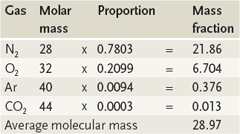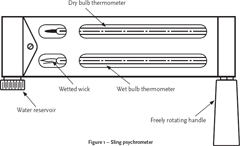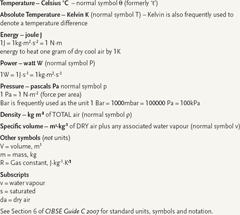# Module 3: The properties of air

This article will look at the basic parameters that combine to provide a psychrometric toolkit. A later article will consider the applications of these properties using the psychrometric chart.Table 1 – The Molecular Mass of Dry Air

Air is normally considered to be a mix of dry air and water vapour. ‘Normal’ dry air itself is mainly nitrogen (N2) and oxygen (O2) with traces of argon (Ar), carbon dioxide (CO2) and other gases (see Table 1). Water vapour (H2O) constitutes about 0.40 per cent of the whole global atmosphere and typically makes up one to four per cent of the mass of the combined air mixture at the Earth’s surface. The molecular actions of the gases combining together have been determined by the Gas Laws and it is assumed that for the work in HVAC these ‘ideal gas law’ relationships are valid. From these laws, the characteristic equation for any gas is given by pV = mRT (see box for definitions of the symbols). This equation allows calculation of the properties of gases under different pressures and temperatures. The value of R, the specific gas constant, may be obtained for any gas by considering the molecular mass, M, of each of the constituent gases where R = R0/M and R0 is the Universal Gas Constant of 8314.7 J·kg-1·mol-1 K-1. For example, using the information from Figure 1, the gas constant for dry air is given by R0/M = 8314.7/28.97 = 287 J.kg-1·K-1. The molecular mass of water vapour is 18 and so the specific gas constant may be obtained from 8314.7/18 = 461 J·kg-1·K-1

These relationships can also be usefully employed to examine the properties of other gas mixtures – as might be found, for example, in controlled specialist environments.

The air dry bulb temperature, θ(°C), is the temperature measured using a standard thermometer that is shielded from any radiant heating or cooling effects. (And, of course, is most frequently sensed using an electrical or electronic device.)

As with any gas, the moving molecules of water vapour in the air exert a pressure. This vapour pressure, Pv (measured in Pa or mbar), is related to the mass of water vapour in the air – the more molecules present, the higher the vapour pressure. If molecules of water vapour were to be continually added to a volume of static air (for example, from a room humidifier) then at some point the air would not be able to support any more molecules – the air is said to be saturated at that point and any further water molecules added will simply condense out. The saturated vapour pressure, Ps, will have been reached – the actual value of this is related to the dry bulb temperature of the air; air with a higher dry bulb temperature will have a greater potential to hold water vapour, and so will have a higher saturated vapour pressure. The vapour pressure of water vapour in humid air compared, as a percentage, with the saturated vapour pressure of air at the same temperature gives the relative humidity, Φ (%) and Φ = Pv / Ps x 100%.

The partial pressure of the water vapour adds to the partial pressures of the other gases in the air, to produce the atmospheric pressure, Pa, so giving Pa = Pda + Ps (this relationship is known as Dalton’s Law) – the standard value of atmospheric pressure is taken as 1013.25mbar (101.325 kPa). The atmospheric pressure will alter with both weather and location and so the relative effect of the vapour pressure will also change.

The mass of the water vapour in 1 kg of dry air is termed the moisture content g (kg/kgda) – this is really a ‘vapour’ content and information from the USA will refer to this as humidity ratio. To avoid errors in writing down small numbers associated with moisture content, the units of g/kgda are frequently used in place of kg/kgda (1g/kgda = 0.001kg/kgda).

As an example of using the relationship PV = mRT, the moisture content, g, of a mixture of dry air and water vapour may be found from a knowledge of the partial pressures in the air. So:
mass water vapour/mass of associated dry air = ms/ma and since PV = mRT, then m = PV/RT, and so (PsV/RsT)/(PaV/RaT) = (Ra/Rs) x (Ps/Pda) = 287/461Ps/(PaPs) = 0.622Ps/(PaPs)Figure 1 – Sling psychrometer

By comparing the moisture content in humid air as a percentage, with the moisture content of saturated air (at the same temperature) the percentage saturation, μ, may be determined where μ = g/gs x 100 per cent. The numerical values of percentage saturation, μ, and relative humidity, Φ, produce similar values and are practically interchangeable for HVAC calculations.

Specific Enthalpy, h (kJ/kgda), is the heat content of humid air per kg dry air – it is relative to the enthalpy dry air at 0°C. Air with higher temperature and/or with moisture will have a positive specific enthalpy (more commonly referred to as simply ‘enthalpy’). The range of conditions likely in HVAC enthalpy can be estimated from h = (1.005θ) + g(1.89θ + 2501); however, more precise results may be obtained using relationships is CIBSE Guide C 2007.

The thermodynamic wet bulb temperature, θ'(°C), is a useful means of determining the moisture content of the air at a particular dry bulb temperature. The wet bulb temperature is the temperature that a sample of air would reach if it had a fine spray of water added to it (the water being at the air dry bulb temperature) without any external heat being added or taken away (this is known as an adiabatic process). Some of the sprayed water will evaporate into the air – the heat to cause that evaporation being taken from the air itself and so the air dry bulb temperature will drop until the point where the air is saturated (with water vapour). At this point the dry bulb temperature = wet bulb temperature, and the air is said to have reached its dew point: if the air dry bulb temperature is then reduced, or more water vapour is added to the air, then condensation will occur.

Practically, the wet bulb temperature is measured with a thermometer with a wetted material wrapped (or more frequently a wick fed from a reservoir of distilled water) around the thermometer’s bulb at the same temperature as the air dry bulb temperature. The evaporation (or drying) of the water from the wick into the surrounding air needs energy, and this is mainly taken from the thermometer’s bulb, so reducing the temperature as read from the stem of the thermometer. The fewer molecules of water vapour in the air (and hence the lower the air vapour pressure), the more molecules of water will be able to evaporate into it from the thermometer’s wetted surface bulb and so the lower the wet bulb temperature.

The difference in the value of the wet bulb temperature compared with the air’s dry bulb temperature, (θ-θ’)(K), is known as the wet bulb depression, (wet bulb is always less than or equal to dry bulb temperature). Dry bulb temperature and wet bulb depression tables (or calculations) can be used to determine the air moisture content. The recording of the wet bulb temperature is commonly undertaken in two ways – the screen wet bulb, θ’sc – and the much more useful sling wet bulb θ’sl. The two values are likely to vary slightly from each other. The screen wet bulb is recorded by a static wet bulb thermometer placed in air (frequently outdoors in a Stevenson’s Screen), and the sling wet bulb is measured where there is air movement across the thermometer’s bulb (ensuring that any evaporated water is carried away from the bulb and so does not locally increase the vapour pressure). These measurements may be undertaken in a number of ways including the Sling Psychrometer (see Figure 1) and the Aspirated Hygrometer.

### Obtaining the properties

CIBSE Guide C (2007) has lists of the values of all the properties of air as described above for various dry bulb temperatures. The guide also includes sets of equations that will allow determination of values for other gases that may be used in spreadsheets or computer programmes to the Characteristic Equation may be used in conjunction with the Gas Laws.

### The psychrometric chart

The parameters described so far are combined to produce the psychrometric chart, which dates back to empirical work undertaken by James Glaisher in Greenwich in the first half of the 19th century. Glaisher had problems reliably determining the wet bulb temperature – these problems arose from the differences between screen and sling wet bulb temperatures. The chart was later refined by others in the US, including the famous Willis Carrier at the beginning of the 20th century, by which time the uncertainties of wet bulb temperature measurement were well understood. The form of the psychrometric chart has changed little from the early versions – the most visually significant alteration being the changes in the units to SI for the CIBSE Chart, see Figure 2.Figure 2 – Standard CIBSE Psychrometric Chart

The chart provides a convenient way of examining the changes in the dependent parameters in air as the air undergoes a process in a space (for example, heating and humidification from occupants) or as it is conditioned in an HVAC system. If any two properties are known, then the others may be read from the chart – so, for example, in the field this would typically be wet bulb and dry bulb temperature measured with a sling psychrometer.

The application of the chart to processes and systems will be explored in a future CPD article.With thanks to Dr Roger Legg for assistance with the material for this article © Tim Dwyer 2009